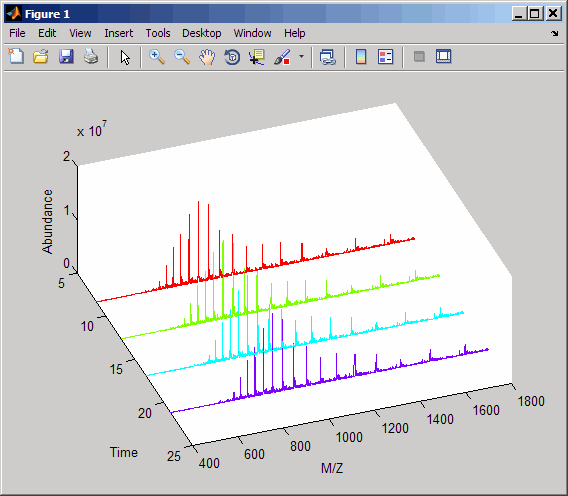# mzcdf2peaks

Convert mzCDF structure to peak list

## Syntax

```[Peaklist, Times] = mzcdf2peaks(mzCDFStruct) ```

## Input Arguments

 `mzCDFStruct` MATLAB® structure containing information from a netCDF file, such as one created by the `mzcdfread` function. Its fields correspond to the variables and global attributes in a netCDF file. If a netCDF variable contains local attributes, an additional field is created, with the name of the field being the variable name appended with `_attributes`. The number and names of the fields will vary, depending on the mass spectrometer software, but typically there are `mass_values` and `intensity_values` fields.

## Output Arguments

 `Peaklist` Either of the following:Two-column matrix, where the first column contains mass/charge (m/z) values and the second column contains ion intensity values.Cell array of peak lists, where each element is a two-column matrix of m/z values and ion intensity values, and each element corresponds to a spectrum or retention time. `Times` Scalar of vector of retention times associated with a liquid chromatography/mass spectrometry (LC/MS) or gas chromatography/mass spectrometry (GC/MS) data set. If `Times` is a vector, the number of elements equals the number of peak lists contained in `Peaklist`.

## Description

```[Peaklist, Times] = mzcdf2peaks(mzCDFStruct)``` extracts peak information from `mzCDFStruct`, a MATLAB structure containing information from a netCDF file, such as one created by the `mzcdfread` function, and creates `Peaklist`, a single matrix or a cell array of matrices containing mass/charge (m/z) values and ion intensity values, and `Times`, a scalar or vector of retention times associated with a liquid chromatography/mass spectrometry (LC/MS) or gas chromatography/mass spectrometry (GC/MS) data set.

`mzCDFStruct` contains fields that correspond to the variables and global attributes in a netCDF file. If a netCDF variable contains local attributes, an additional field is created, with the name of the field being the variable name appended with `_attributes`. The number and names of the fields will vary, depending on the mass spectrometer software, but typically there are `mass_values` and `intensity_values` fields.

## Examples

In the following example, the file `results.cdf` is not provided.

1. Use the `mzcdfread` function to read a netCDF file into the MATLAB software as a structure. Then extract the peak information from the structure.

```mzcdf_struct = mzcdfread('results.cdf'); [peaks,time] = mzcdf2peaks(mzcdf_struct) peaks = [7008x2 single] [7008x2 single] [7008x2 single] [7008x2 single] time = 8.3430 12.6130 16.8830 21.1530 ```
2. Create a color map containing a color for each peak list (retention time).

```colors = hsv(numel(peaks)); ```
3. Create a 3-D figure of the peaks and add labels to it.

```figure hold on for i = 1:numel(peaks) t = repmat(time(i),size(peaks{i},1),1); plot3(t,peaks{i}(:,1),peaks{i}(:,2),'color',colors(i,:)) end view(70,60) xlabel('Time') ylabel(mzcdf_struct.mass_axis_label) zlabel(mzcdf_struct.intensity_axis_label) ```